Support

Apps

•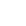My Wallet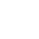My Order
•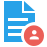My Profile
•My Connections
•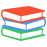My Books
•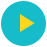My Videos
•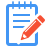My Tests
•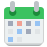My Calender
•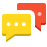My Messages
•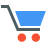My Shopping Cart
•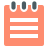My Orders
•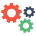Account Settings
•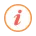Help

# Book Details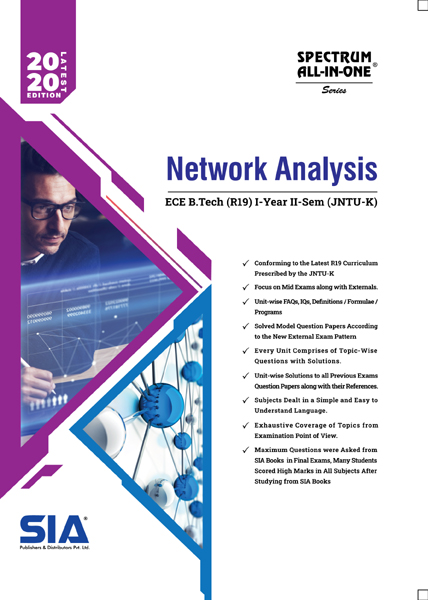# Network Analysis (JNTU-K)

 Course Code : B.Tech I-II (ECE) R19 Author : SIA PUBLISHERS University : JNTU Kakinada Regulation : 2020 Categories : Engineering Format :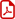PDF (DRM Protected) Type : eBook

Rs.249 Rs.137 Rs.45% off

Description :

SYLLABUS

UNIT-I

INTRODUCTION TO ELECTRICAL CIRCUITS Network elements classification, Electric charge and current, Electric energy and potential, Resistance parameter – Series and parallel combination, Inductance parameter – Series and parallel combination, Capacitance parameter – Series and parallel combination. Energy sources: Ideal, Non-ideal, Independent and dependent sources, Source transformation, Kirchoff’s laws, Mesh analysis and Nodal analysis problem solving with resistances only including dependent sources also. A.C FUNDAMENTALS AND NETWORK TOPOLOGY Definitions of terms associated with periodic functions: Time period, Angular velocity and frequency, RMS value, Average value, Form factor and peak factor-problem solving, Phase angle, Phasor representation, Addition and subtraction of phasors, Mathematical representation of sinusoidal quantities, Explanation with relevant theory, Problem solving. Principal of Duality with examples. NETWORK TOPOLOGY Definitions of branch, Node, Tree, Planar, Non-planar graph, Incidence matrix, Basic tie set schedule, Basic cut set schedule.

UNIT-II

TRANSIENTS First order differential equations, Definiation of time constants, R-L circuit, R-C circuit with DC excitation, Evaluating initial conditions procedure, Second order differential equations, Homogeneous, Non-homogenous, Problem solving using R-L-C elements with DC excitation and AC excitation, Response as related to s-plane rotation of roots. Solution using Laplace transform method.

UNIT-III

STEADY STATE ANALYSIS OF A.C CIRCUITS Impedance concept, Phase angle, Series R-L, R-C, R-L-C circuits problem solving. Complex impedance and phasor notation for R-L, R-C, R-L-C problem solving using mesh and nodal analysis, Star-Delta conversion, Problem solving. COUPLED CIRCUITS Coupled circuits: Self inductance, Mutual inductance, Coefficient of coupling, Analysis of coupled circuits, Natural current, Dot rule of coupled circuits, Conductively coupled equivalent circuits – Problem solving.

UNIT-IV

RESONANCE Introduction, Definition of Q, Series resonance, Bandwidth of series resonance, Parallel resonance, Condition for maximum impedance, Current in anti resonance, Bandwidth of parallel resonance, General case-resistance present in both branches, Anti resonance at all frequencies. NETWORK THEOREMS Thevenin’s, Norton’s, Milliman’s, Reciprocity, Compensation, Substitution, Superposition, Max power transfer, Tellegens-problem solving using dependent sources also.

UNIT-V

TWO-PORT NETWORKS Relationship of two port networks, Z-parameters, Y-parameters, Transmission line parameters, h-parameters, Inverse h-parameters, Inverse transmission line parameters, Relationship between parameter sets, Parallel connection of two port networks, Cascading of two port networks, Series connection of two port networks, Problem solving including dependent sources also.

Note : Network Analysis (JNTU-K) B.Tech I-II (ECE) R19

##### Publisher Detail:

Publisher Name: SIA Publishers and Distributors (P) Ltd.

Contact Email Id: msultan@siaedugroup.com